Download Presentation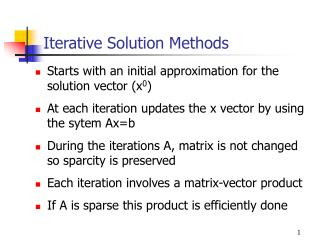Iterative Solution Methods

# Iterative Solution Methods - PowerPoint PPT Presentation

Iterative Solution Methods. Starts with an initial approximation for the solution vector (x 0 ) At each iteration updates the x vector by using the sytem Ax=b During the iterations A, matrix is not changed so sparcity is preserved Each iteration involves a matrix-vector productI am the owner, or an agent authorized to act on behalf of the owner, of the copyrighted work described.
Download Presentation## Iterative Solution Methods

An Image/Link below is provided (as is) to download presentation

Download Policy: Content on the Website is provided to you AS IS for your information and personal use and may not be sold / licensed / shared on other websites without getting consent from its author.While downloading, if for some reason you are not able to download a presentation, the publisher may have deleted the file from their server.

- - - - - - - - - - - - - - - - - - - - - - - - - - E N D - - - - - - - - - - - - - - - - - - - - - - - - - -
Presentation Transcript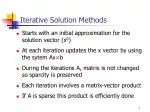Iterative Solution Methods
• Starts with an initial approximation for the solution vector (x0)
• At each iteration updates the x vector by using the sytem Ax=b
• During the iterations A, matrix is not changed so sparcity is preserved
• Each iteration involves a matrix-vector product
• If A is sparse this product is efficiently done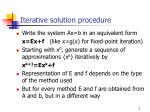Iterative solution procedure
• Write the system Ax=b in an equivalent form

x=Ex+f (like x=g(x) for fixed-point iteration)

• Starting with x0, generate a sequence of approximations {xk} iteratively by

xk+1=Exk+f

• Representation of E and f depends on the type of the method used
• But for every method E and f are obtained from A and b, but in a different way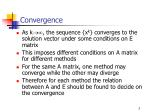Convergence
• As k, the sequence {xk} converges to the solution vector under some conditions on E matrix
• This imposes different conditions on A matrix for different methods
• For the same A matrix, one method may converge while the other may diverge
• Therefore for each method the relation between A and E should be found to decide on the convergence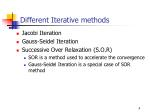Different Iterative methods
• Jacobi Iteration
• Gauss-Seidel Iteration
• Successive Over Relaxation (S.O.R)
• SOR is a method used to accelerate the convergence
• Gauss-Seidel Iteration is a special case of SOR method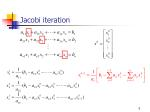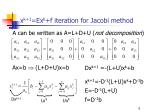Dxk+1

xk+1=Exk+f iteration for Jacobi method

A can be written as A=L+D+U (not decomposition)

Ax=b  (L+D+U)x=b

Dxk+1 =-(L+U)xk+b

xk+1=-D-1(L+U)xk+D-1b

E=-D-1(L+U)

f=D-1b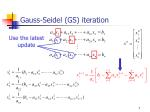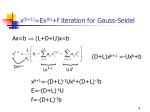Dxk+1

x(k+1)=Ex(k)+f iteration for Gauss-Seidel

Ax=b  (L+D+U)x=b

(D+L)xk+1 =-Uxk+b

xk+1=-(D+L)-1Uxk+(D+L)-1b

E=-(D+L)-1U

f=-(D+L)-1b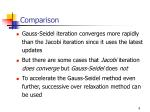Comparison
• Gauss-Seidel iteration converges more rapidly than the Jacobi iteration since it uses the latest updates
• But there are some cases that Jacobi iteration does converge but Gauss-Seidel does not
• To accelerate the Gauss-Seidel method even further, successive over relaxation method can be used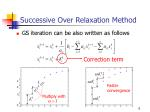Correction term

Multiply with

Successive Over Relaxation Method
• GS iteration can be also written as follows

Faster

convergenceSOR

1<<2 over relaxation (faster convergence)

0<<1 under relaxation (slower convergence)

There is an optimum value for 

Find it by trial and error (usually around 1.6)x(k+1)=Ex(k)+f iteration for SOR

Dxk+1=(1-)Dxk+b-Lxk+1-Uxk

(D+ L)xk+1=[(1-)D-U]xk+b

E=(D+ L)-1[(1-)D-U]

f= (D+ L)-1bThe Conjugate Gradient Method
• Converges if A is a symmetric positive definite matrix
• Convergence is fasterSubstitute this into

Convergence of Iterative Methods

Define the solution vector as

Define an error vector asConvergence of Iterative Methods

power

iteration

The iterative method will converge for any initial

iteration vector if the following condition is satisfied

Convergence conditionNorm of a vector

A vector norm should satisfy these conditions

Vector norms can be defined in different forms as long as the norm definition satisfies these conditionsCommonly used vector norms

Sum norm or ℓ1 norm

Euclidean norm or ℓ2 norm

Maximum norm or ℓ normNorm of a matrix

A matrix norm should satisfy these conditions

Important identitiyCommonly used matrix norms

Maximum column-sum norm or ℓ1 norm

Spectral norm or ℓ2 norm

Maximum row-sum norm or ℓ norm17

13

15

16

19

10

Example
• Compute the ℓ1 and ℓ norms of the matrixConvergence condition

Express E in terms of modal matrix P and 

:Diagonal matrix with eigenvalues of E on the diagonalis a sufficient condition for convergence

Sufficient condition for convergence

If the magnitude of all eigenvalues of iteration matrix

E is less than 1 than the iteration is convergent

It is easier to compute the norm of a matrix than to compute its eigenvaluesConvergence of Jacobi iteration

Evaluate the infinity(maximum row sum) norm of E

Diagonally dominant matrix

If A is a diagonally dominant matrix, then Jacobi iteration converges for any initial vectorStopping Criteria
• Ax=b
• At any iteration k, the residual term is

rk=b-Axk

• Check the norm of the residual term

||b-Axk||

• If it is less than a threshold value stopExample 1 (Jacobi Iteration)

Diagonally dominant matrixExample 1 continued...

Matrix is diagonally dominant, Jacobi iterations are convergingExample 2

The matrix is not diagonally dominantExample 2 continued...

The residual term is increasing at each iteration, so the iterations are diverging.

Note that the matrix is not diagonally dominantConvergence of Gauss-Seidel iteration
• GS iteration converges for any initial vector if A is a diagonally dominant matrix
• GS iteration converges for any initial vector if A is a symmetric and positive definite matrix
• Matrix A is positive definite if

xTAx>0 for every nonzero x vectorPositive Definite Matrices
• A matrix is positive definite if all its eigenvalues are positive
• A symmetric diagonally dominant matrix with positive diagonal entries is positive definite
• If a matrix is positive definite
• All the diagonal entries are positive
• The largest (in magnitude) element of the whole matrix must lie on the diagonalNot positive definite

Largest element is not on the diagonal

Not positive definite

All diagonal entries are not positive

Positive Definitiness Check

Positive definite

Symmetric, diagonally dominant, all diagonal entries are positivePositive Definitiness Check

A decision can not be made just by investigating the matrix.

The matrix is diagonally dominant and all diagonal entries are positive but it is not symmetric.

To decide, check if all the eigenvalues are positiveJacobi iteration

Example 1 continued...

When both Jacobi and Gauss-Seidel iterations converge, Gauss-Seidel converges fasterConvergence of SOR method
• If 0<<2, SOR method converges for any initial vector if A matrix is symmetric and positive definite
• If >2, SOR method diverges
• If 0<<1, SOR method converges but the convergence rate is slower (deceleration) than the Gauss-Seidel method.Operation count
• The operation count for Gaussian Elimination or LU Decomposition was 0 (n3), order of n3.
• For iterative methods, the number of scalar multiplications is 0 (n2) at each iteration.
• If the total number of iterations required for convergence is much less than n, then iterative methods are more efficient than direct methods.
• Also iterative methods are well suited for sparse matrices